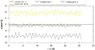# Any idea about how determine body movement upun the accelerations?

niko2000
Any idea about how determine body movement upon the accelerations?

Hi,

I am trying to implement an algorithm which would determine the person's activity upon the received acceleration measurement data.

I am measuring the accelerations using accelerometer sensors on mobile phones and send the data to the server where I process the measurement and try to determine the type of person's movement. The key idea is to develop the software which would automatically call the emergency if the person would fall (useful for old people).

So far I was trying to use Fourier transform and measure the frequency components but this by itself is not accurate enough. Enclosed I am sending an example chart of walk.

In case of fall it is easy to guess what happened by watching the chart but the goal is to implement an automatic detection.

I would really appreciate if you could share your idea about analyzing the patterns and determine what happened. It would be great if a computer could determine for example if the person is walking, running or if a person fell.

Thanks for help!

Niko

#### Attachments

•walk.jpg
33.2 KB · Views: 381
Last edited:

Homework Helper
First, the good news- you can treat the x, y, and z components separately.

Now, the bad news- if your acceleration data is not constant, you will need to do a numerical integration of the data.

Given a(t) as the acceleration for a particular component, $v(t)= \int_{T_0}^T a(t)dt+ v_0)$ is the speed at time T in that direction assuming speed v0 at time T0 in that direction.

$p(t)= \int_{T_0}^T v(t)dt+ p_0$ is the component of position itself at time T given component p0 at time T0.